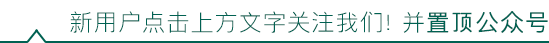# 7 种 PHP 获取文件扩展名的方法PHP中获取文件扩展名的方法

$file = 'x.y.z.png'; echo substr(strrchr($file, '.'), 1);


echo substr($file, strrpos($file, '.')+1);


$arr = explode('.',$file);
echo $arr[count($arr)-1];


$file = 'x.y.z.png';$arr = explode('.', $file); echo end($arr);  //end()返回数组的最后一个元素


$file = 'x.y.z.png'; echo strrev(explode('.', strrev($file)));


.$file = 'x.y.z.png'; echo pathinfo($file)['extension'];


[dirname]
[basename]
[extension]


.$file = 'x.y.z.png'; echo pathinfo($file, PATHINFO_EXTENSION)©️2019 CSDN 皮肤主题: 大白 设计师: CSDN官方博客